# Colleges with the lowest SAT scores in Massachusetts

Top 10 colleges in Massachusetts with the lowest SAT scores
Looking for the colleges with the lowest SAT scores in Massachusetts? Well you're in luck! We've compiled a national college database and have created a list of the top 10 universities with the lowest SAT scores in Massachusetts below. If you are not a good test taker or worried about your test scores, this list is for you. These are the schools whose applicants had the lowest average SAT scores in Massachusetts, which means that you can get into these colleges with a lower SAT score. We also include each college's ACT scores and acceptance rate so that you can see where you would have the easiest time getting in. Read on to find out more.

## Mount Ida College SAT scores

The average SAT score for Mount Ida College is 950.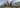The average SAT score of 950 breaks down into:

• SAT math: 470

The average ACT score for Mount Ida College is 17 and their acceptance rate is 63%.

## Curry College SAT scores

The average SAT score for Curry College is 1026.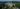The average SAT score of 1026 breaks down into:

• SAT math: 510

The average ACT score for Curry College is 21 and their acceptance rate is 92.7%.

## Emmanuel College SAT scores

The average SAT score for Emmanuel College is 1030.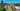The average SAT score of 1030 breaks down into:

• SAT math: 510

The average ACT score for Emmanuel College is 20 and their acceptance rate is 22%.

## Wheelock College SAT scores

The average SAT score for Wheelock College is 1030.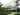The average SAT score of 1030 breaks down into:

• SAT math: 500

The average ACT score for Wheelock College is 20 and their acceptance rate is 95%.

## Framingham State University SAT scores

The average SAT score for Framingham State University is 1050.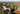The average SAT score of 1050 breaks down into:

• SAT math: 520

The average ACT score for Framingham State University is 21 and their acceptance rate is 73.3%.

## Bridgewater State University SAT scores

The average SAT score for Bridgewater State University is 1070.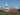The average SAT score of 1070 breaks down into:

• SAT math: 530

The average ACT score for Bridgewater State University is 22 and their acceptance rate is 90.1%.

## Elms College SAT scores

The average SAT score for Elms College is 1070.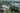The average SAT score of 1070 breaks down into:

• SAT math: 540

The average ACT score for Elms College is 21 and their acceptance rate is 67.5%.

## Fitchburg State University SAT scores

The average SAT score for Fitchburg State University is 1072.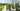The average SAT score of 1072 breaks down into:

• SAT math: 540

The average ACT score for Fitchburg State University is 21 and their acceptance rate is 86.9%.

## Salem State University SAT scores

The average SAT score for Salem State University is 1080.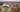The average SAT score of 1080 breaks down into:

• SAT math: 530

The average ACT score for Salem State University is 21 and their acceptance rate is 71.7%.

## Suffolk University SAT scores

The average SAT score for Suffolk University is 1086.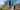The average SAT score of 1086 breaks down into:

• SAT math: 540